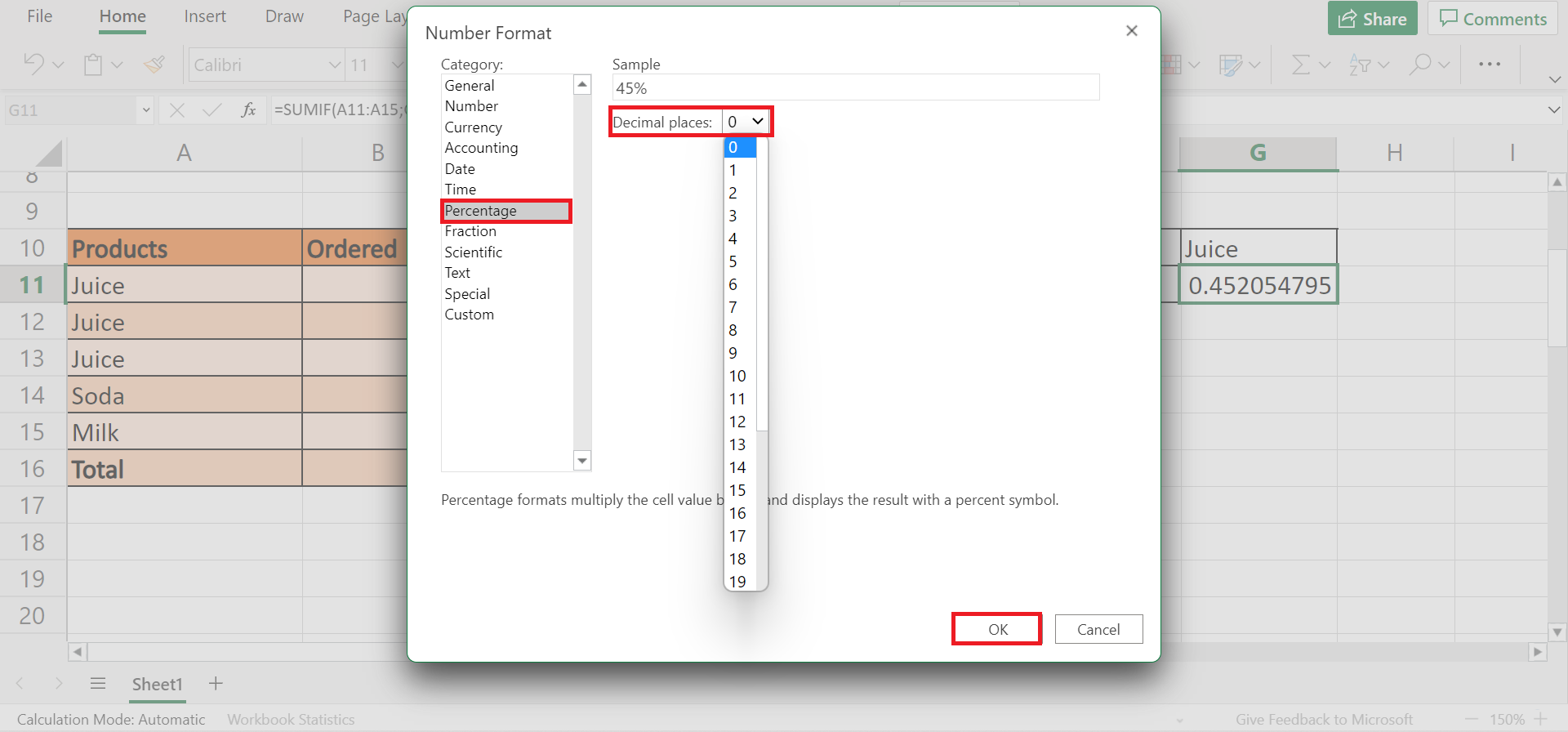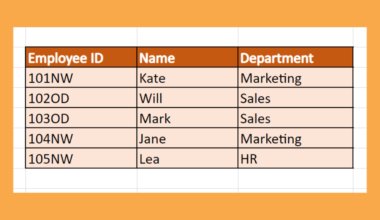# How to Calculate Percentage as a Proportion in Excel

This guide will discuss how to calculate percentage as a proportion in Excel

In Excel, calculating the percentage is simple. It only takes a few steps and focuses on basic arithmetic.

In mathematics, a proportion is essentially a comparison between two numbers. So it is when two ratios are equal to each other. Simply put, it is a share or part in comparison or in relation to a whole group or number.

A percentage is part of a 100. For example, 50 out 0f 100 or in percent format, 50%. So what is the relationship between percent and proportion?

First, they both pertain to a part of a whole. When we multiply a proportion by 100, we get the percentage of the parts that were taken from the whole. Similarly, we get the proportion by multiplying the percentage by the whole.

We will tackle two ways to calculate percentages as proportions in Excel depending on the data set. The first is simply dividing the part or sample value by the whole or total value. And second is using the `SUMIF` function.

Let’s take a scenario where you must calculate a percentage as a proportion in Excel.

Suppose you are a teacher who wants to calculate the percentage score of the student’s exam results. So you want to know what is the percentage score of a student who scores 85 out of 100 on an exam.

In this example, you can get the percentage as a proportion by dividing 85 by 100. Furthermore, you can do this task quickly and easily in Excel, especially when handling large amounts of data.

## The Anatomy of the SUMIF Function

The syntax or the way we write the `SUMIF` function is as follows:

`=SUMIF(range;criteria;[sum_range])`

Let’s take each term and understand what they mean:

• = the equal sign is how we start any equation or function in Excel.
• SUMIF() is our `SUMIF` function. This function performs a sum calculation of a range based on the given condition.
• criteria refer to the conditions set. This can check whether the value is greater than, less than, or equal to something. In this case, we use it to look for a specific product to calculate.
• sum_range is an optional argument. This is the range where the `SUMIF` function calculates the sum.

Awesome! Let’s dive into a real example of calculating the percentage as a proportion in Excel.

## A Real Example of Calculating Percentage as a Proportion in Excel

Let’s take a look at our sample data. For instance, we want to find the percentage of each product ordered out of the total products ordered.In this case, we have the total number of products ordered, which makes this calculation easy. The formula for calculating percentage as a proportion in Excel is simply `part/total = percentage`

Essentially, we only need to divide the number of the specific product ordered by the total number of products ordered.

Now let’s look at another situation. For instance, we want to calculate the percentage as a proportion of specific products only. In this case, we only want to know the percentage of Juices ordered out of the total products ordered.In this case, we will make use of the `SUMIF` function. First, we need to input the criteria, which in this example would be juice. Then, we need to select the range of the products ordered. Finally, divide by the total products ordered. And tada! We can get the percentage of Juice products ordered.

Now let’s learn the step-by-step process of how to perform this calculation to apply to your work.

## How to Calculate Percentage as a Proportion in Excel

This section will discuss the steps on how to calculate the percentage as a proportion in Excel.

1. First, type in the = equal sign to start the calculation. Then, select the cell containing the part or proportion you want to calculate. In this case, we select B2. Finally, divide that by the total number of ordered products which is in B7.Also, we will enclose B7 to turn it into an absolute cell reference. This locks it as our denominator when we drag down the formula. So the entire formula would be `=B2/\$B\$7`.

2. Second, drag down the formula from B2 to the entire column to apply the same formula.3. Next, we need to change the result from decimal to percentage. To do this, go to Home and click the Number Format dropdown menu. Then, select the Percentage.4. If there are decimal places on the percentages, we can simply remove this. First, select the percentage column and right-click. Then, click on Number Format.5. On the Number Format window, go to the Percentage category. And change the Decimal places to zero. Finally, click OK to apply the changes.6. Now, let’s calculate the percentage of specific products only. First, let’s create a table where we will input the result. Specify the product you are using, which in this case is Juice. Also, we will use this as a cell reference.7. Next, type in ‘=SUMIF’ to start the function. Then, select the range containing the products which are A11:A15, and select the cell containing the product you are looking for, which is G10. And this will be our criteria.

Finally, select the range containing the number of orders which is B11:B15, and divide everything by the total number of ordered products. So the entire formula would be `=SUMIF(A11:A15;G10;B11:B15)/\$B\$16`.8. Again, we need to change the result from decimal to a percentage. So right-click on the decimal and select Number Format. Then, select the Percentage category and change the Decimal places to zero. Lastly, click OK to apply changes.9. And tada! We have successfully calculated the percentage as a proportion in Excel.Great! That’s pretty much it. You have learned how to calculate the percentage as a proportion in Excel using the basic formula and `SUMIF` function.

Are you interested in learning more about what Excel can do? You can now use the various other Microsoft Excel formulas available to create great worksheets that work for you. Make sure to subscribe to our newsletter to be the first to know about the latest guides and tutorials from us.Our goal this year is to create lots of rich, bite-sized tutorials for Google Sheets users like you. If you liked this one, you'll love what we are working on! Readers receive ✨ early access ✨ to new content.

##### You May Also Like## How to Rank Non-Continuous Range in Excel

This guide will explain how you can use the RANK function to rank a non-continuous range in Excel.…This guide will explain how to use Google Bard with Google Sheets and Microsoft Excel. Bard is an…## How to Insert or Display Named Range on Another Sheet in Excel

This guide will show you how to insert or display a named range on another sheet in Excel.…## How to Use Wildcard in FILTER Function in Excel

This guide will explain how to use wildcards in the FILTER function in Excel. The rules for using…